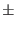Next: Junction Field-Effect Transistors (JFETs) Up: Semiconductor Devices Previous: Bipolar Junction Transistors (BJTs)   Contents   Index

## BJT Models (both NPN and PNP)

Type Names: npn, pnp

The bipolar junction transistor model in WRspice is an adaptation of the integral charge control model of Gummel and Poon. This modified Gummel-Poon model extends the original model to include several effects at high bias levels. The model will automatically simplify to the simpler Ebers-Moll model when certain parameters are not specified. The parameter names used in the modified Gummel-Poon model have been chosen to be more easily understood by the program user, and to reflect better both physical and circuit design thinking.

The dc model is defined by the parameters is, bf, nf, ise, ikf, and ne which determine the forward current gain characteristics, is, br, nr, isc, ikr, and nc which determine the reverse current gain characteristics, and vaf and var which determine the output conductance for forward and reverse regions. Three ohmic resistances rb, rc, and re are included, where rb can be high current dependent. Base charge storage is modeled by forward and reverse transit times, tf and tr, the forward transit time tf being bias dependent if desired, and nonlinear depletion layer capacitances which are determined by cje, vje, and mje for the B-E junction, cjc, vjc, and mjc for the B-C junction, and cjs, vjs, and mjs for the C-S (Collector-Substrate) junction. The temperature dependence of the saturation current, is, is determined by the energy gap, eg, and the saturation current temperature exponent, xti. Additionally base current temperature dependence is modeled by the beta temperature exponent xtb in the new model. The values specified are assumed to have been measured at the temperature tnom, which can be specified on the .options line or overridden by a specification on the .model line.

The BJT parameters used in the modified Gummel-Poon model are listed below. The parameter names used in earlier versions of SPICE2 are still accepted. The parameters marked with an asterisk in the area column scale with the area parameter given in the device line.

There is also a level=4 BJT model which uses the VBIC equation set, as used in the NGspice-17 simulator. This model is documented elsewhere.

 name BJT Model Parameters area parameter units default example is * transport saturation current A 1.0e-16 1.0e-15 bf ideal maximum forward beta - 100 100 nf forward current emission coefficient - 1.0 1 vaf forward Early voltage V infinite 200 ikf * corner for forward beta high current roll-off A infinite 0.01 ise * B-E leakage saturation current A 0 1.0e-13 ne B-E leakage emission coefficient - 1.5 2 br ideal maximum reverse beta - 1 0.1 nr reverse current emission coefficient - 1 1 var reverse Early voltage V infinite 200 ikr * corner for reverse beta high current roll-off A infinite 0.01 isc * B-C leakage saturation current A 0 1.0e-13 nc B-C leakage emission coefficient - 2 1.5 rb * zero bias base resistance0 100 ikb * current where base resistance falls halfway to its min value A infinite 0.1 rbm * minimum base resistance at high currentsrb 10 re * emitter resistance0 1 rc * collector resistance0 10 cje * B-E zero-bias depletion capacitance F 0 2pf vje B-E built-in potential V 0.75 0.6 mje B-E junction exponential factor - 0.33 0.33 tf ideal forward transit time S 0 0.1ns xtf coefficient for bias dependence of tf - 0 - vtf voltage describing VBC dependence of tf V infinite - itf * high-current parameter for effect on tf A 0 - ptf excess phase at freq=1.0/(tf . 2) Hz deg 0 - cjc * B-C zero-bias depletion capacitance F 0 2pf vjc B-C built-in potential V 0.75 0.5 mjc B-C junction exponential factor - 0.33 0.5 xcjc fraction of B-C depletion capacitance connected to internal base node - 1 - tr ideal reverse transit time S 0 10ns cjs * zero-bias collector-substrate capacitance F 0 2pf vjs substrate junction built-in potential V 0.75 - mjs substrate junction exponential factor - 0 0.5 xtb forward and reverse beta temperature exponent - 0 - eg energy gap for temperature effect on is eV 1.11 - xti temperature exponent for effect on is - 3 - kf flicker-noise coefficient - 0 - af flicker-noise exponent - 1 - fc coefficient for forward-bias depletion capacitance formula - 0.5 - tnom parameter measurement temperature C 25 50Next: Junction Field-Effect Transistors (JFETs) Up: Semiconductor Devices Previous: Bipolar Junction Transistors (BJTs)   Contents   Index
Stephen R. Whiteley 2021-01-27